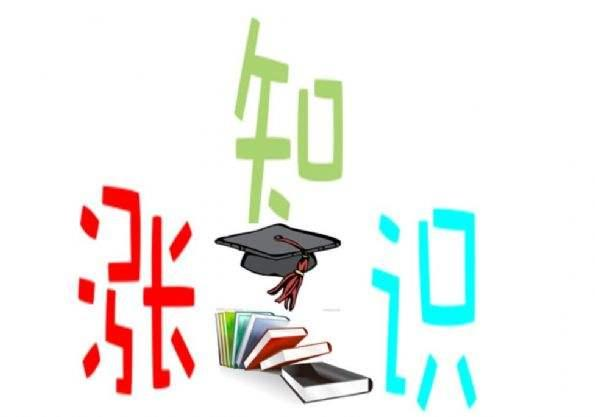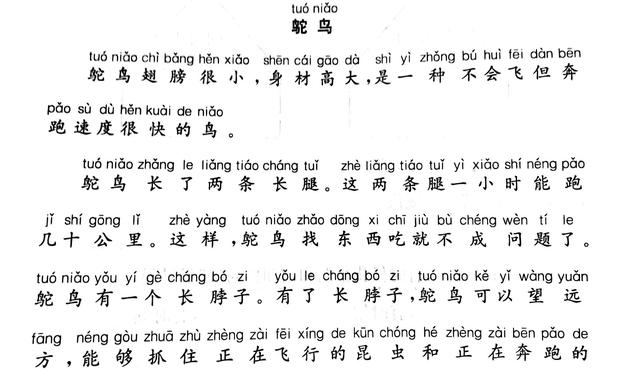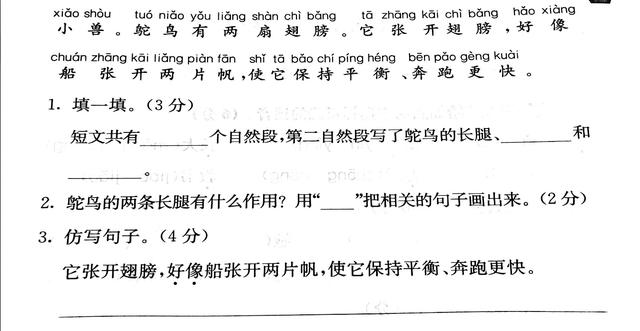# 上海沪教版二年级本周必会知识点，明天就考（语数英综合）

2021-01-04 108 ℃Sweet House 二年级第六周巩固内容① 乘法口诀不熟的要巩固。（活动，乘法口诀接龙）

② 看进度情况决定是否讲除法。(PPT上对于除法的理解)

③ 口算加强，习题巩固，应用理解。

1.口算

4×5=（ ）×2 3×8=（ ）×4 2×8=（ ）×4 6×8= 4×9= 10×8= 0×7＋9= 19-2×0= 39＋18= 44-17＋16= （ ）×2=8 6×8-9=

24=（ ）×8 （ ）×8=0 16＋（ ）=49 （ ）＋18=37

（ ）-16=45 （ ）＋7=66 16＋7×8= 56-4×9=

2. 画一画

3.有两个鱼缸，每个鱼缸有6条鱼，一共有几条鱼？

① 常规课本单词。

② 综合知识汇总考查并详解

1.（ ）I’m Supergirl.

A.a B. / C. an

2.（ ）She has elephant.

A.a B./ C.an

3.（ ）Can you rope？

A. play B.skip C.ride

4.（ ）—What can do？—You can sing a song.

A.you B. I C. she

5.（ ）— can supergrirl do？—She can fly.

A. How B. Who C. What

6. （ ）I can read，but I write.

A. can B.can’t C. am

7.（ ）—Can you draw, Alice？—

A. Yes，she can. B. No，she can’t. C. No，I can’t.

8. （ ）What can you do，Danny？

A. He can swim. B. I can skip.

9.（ ）Supergirl can swim，but she write.

A. can B. can’t

10. （ ）The boy can read a .

A. bicycle B. book

11. （ ）Can you dance，Danny？

A. Yes，I can. B. No, I can’t.